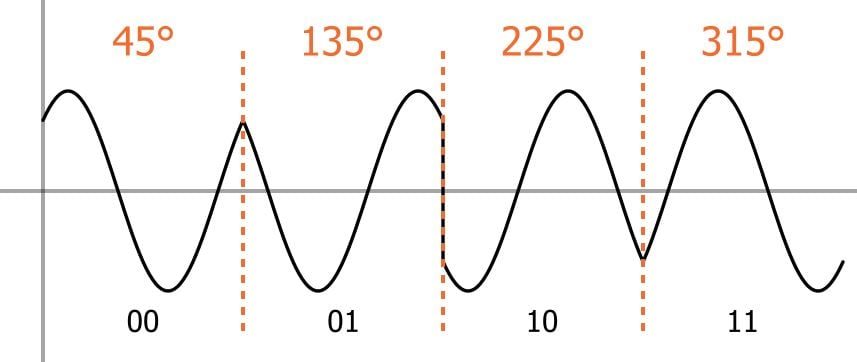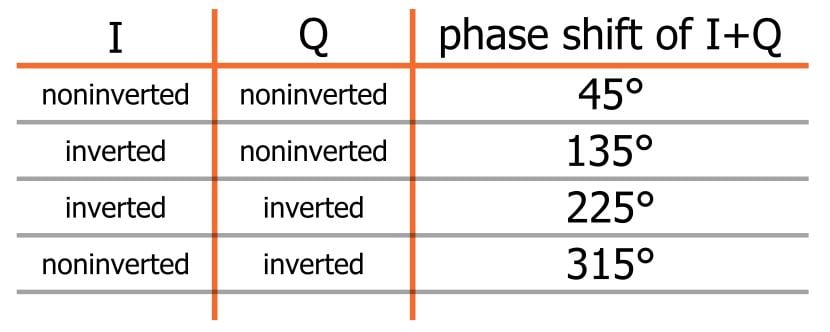Technical Article

# Understanding Quadrature Phase Shift Keying (QPSK) Modulation

August 17, 2016 by Robert Keim

## This technical brief covers the basic characteristics of a digital modulation scheme known as quadrature phase shift keying.

This technical brief covers the basic characteristics of a digital modulation scheme known as quadrature phase shift keying.

In the world of wired electronics, analog signals exhibit continuous variations whereas digital signals assume (ideally) one of two discrete states. This distinction can be extended to systems that transmit data via electromagnetic radiation instead of electric current traveling through wires.

When used for analog signals, frequency modulation and amplitude modulation lead to continuous variations in the frequency or amplitude of a carrier wave. When modulation techniques are used for digital communication, the variations applied to the carrier are restricted according to the discrete information being transmitted.

Examples of common digital modulation types are OOK (on/off keying), ASK (amplitude shift keying), and FSK (frequency shift keying). These schemes cause the carrier to assume one of two possible states depending on whether the system must transmit a binary 1 or a binary 0; each discrete carrier state is referred to as a symbol.

Quadrature phase shift keying (QPSK) is another modulation technique, and it’s a particularly interesting one because it actually transmits two bits per symbol. In other words, a QPSK symbol doesn’t represent 0 or 1—it represents 00, 01, 10, or 11.

This two-bits-per-symbol performance is possible because the carrier variations are not limited to two states. In ASK, for example, the carrier amplitude is either amplitude option A (representing a 1) or amplitude option B (representing a 0). In QPSK, the carrier varies in terms of phase, not frequency, and there are four possible phase shifts.

We can intuitively determine what these four possible phase shifts should be: First we recall that modulation is only the beginning of the communication process; the receiver needs to be able to extract the original information from the modulated signal. Next, it makes sense to seek maximum separation between the four phase options, so that the receiver has less difficulty distinguishing one state from another. We have 360° of phase to work with and four phase states, and thus the separation should be 360°/4 = 90°. So our four QPSK phase shifts are 45°, 135°, 225°, and 315°.(Note: The phase-shift-to-digital-data correspondence shown above is a logical though arbitrary choice; as long as the transmitter and receiver agree to interpret phase shifts in the same way, different correspondence schemes can be used.)

There’s another reason why it makes sense to choose 45°, 135°, 225°, and 315°: they are easily generated using I/Q modulation techniques because summing I and Q signals that are either inverted or noninverted results in these four phase shifts. The following table should clarify this:Compared to modulation schemes that transmit one bit per symbol, QPSK is advantageous in terms of bandwidth efficiency. For example, imagine an analog baseband signal in a BPSK (binary phase shift keying) system. BPSK uses two possible phase shifts instead of four, and thus it can transmit only one bit per symbol. The baseband signal has a certain frequency, and during each symbol period, one bit can be transmitted. A QPSK system can use a baseband signal of the same frequency, yet it transmits two bits during each symbol period. Thus, its bandwidth efficiency is (ideally) higher by a factor of two.•NobleBrutus November 21, 2018
•RK37 November 26, 2018